# Gm of Differential Amplifiers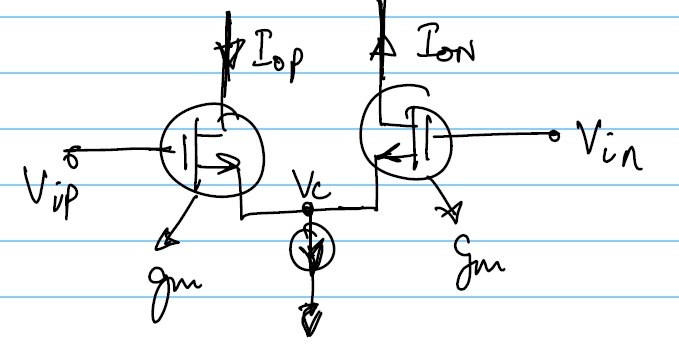A basic differential amplifier input stage looks like:Vip, Vin, Iop and Ion are small signal quantities
NOTE: \$I_{op}=I_{on}\$

\$G_m = I_{out}/V_{in}\$ \$V_{in}\$ is always defined as \$=V_{ip}-V_{in}\$

Now we need to define what is \$I_{out}\$

CASE 1: Output is differntial so the output current \$I_{out}=I_{op}=I_{on}\$

This is because to produce a differential output over symmetric resistances: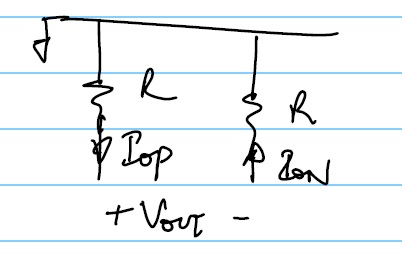\$\$V_{out} = -I_{op}R-I_{on}R = -2I_{out}R\$\$

Thus we get the differential voltage properly

\$\$ ∴ G_m=I_{op}/{V_{ip}-V_{in}} = I_{op}/{(V_{ip}-V_c)-(V_{in}-V_c)}\$\$
\$\$ ⇒ G_m=I_{op}/{I_{op}/g_m-(-I_{on}/g_m)} = I_{op}/{ {I_{op}+I_{op}} /g_m} = g_m/2\$\$

CASE 2: Output is single ended by active mirroring as shown below: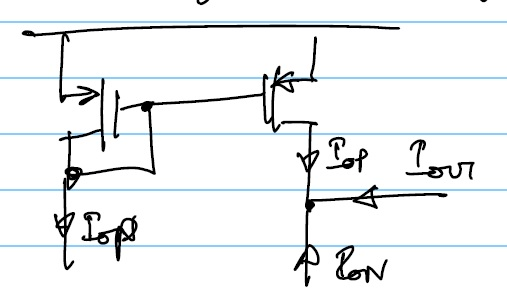\$\$I_{out}=-(I_{op}+I_{on}) = -2I_{op}\$\$
\$\$ ∴ G_m=2 I_{op}/{I_{op}/g_m-(-I_{on}/g_m)} = -2I_{op}/{2I_{op}} g_m = -g_m\$\$

• Thus the OTA which produces single ended outputs by active mirroring have \$G_m = g_m\$
• The OTA which produces differential output or just takes the current from 1 branch of the diffamp have \$G_m=g_m/2\$
where \$g_m\$ = Transconductance of the differential pair element

For Example: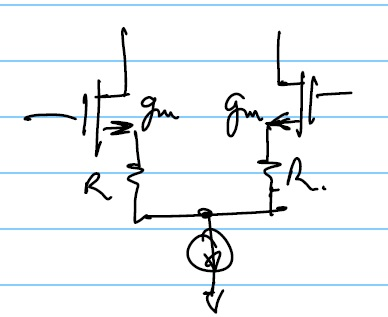CASE 1: \$\$G_m=g_m/{2(1+g_mR)}\$\$
CASE 2: \$\$G_m=g_m/{1+g_mR}\$\$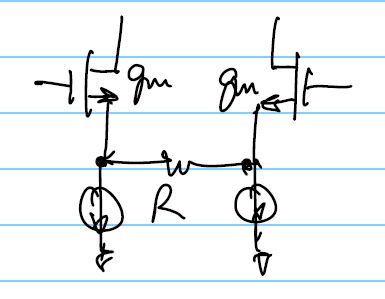CASE 1: \$\$G_m=g_m/{2(1+g_mR/2)}\$\$
CASE 2: \$\$G_m=g_m/{1+g_mR/2}\$\$